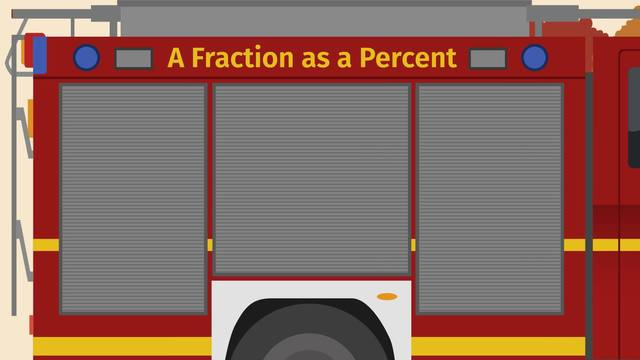# A Fraction as a PercentRating

Be the first to give a rating!

The authorsChris S.

## Basics on the topicA Fraction as a Percent

After this lesson you will be able to convert fractions to percents and back again, using visual models and double number lines.

The lesson begins with a review of the definition of percent, which leads to a method for writing any percent as a fraction over 100. It continues by applying this method to solve real world problems, with the help of visual models and your knowledge of equivalent fractions.

Learn how to convert fractions to percents and back again, by helping Olivia battle wildfires!

This video includes key concepts, notation, and vocabulary such as: percent (a quantity out of 100); equivalent fractions (two fractions which represent the same proportional relationship); and double number line (a visual representation of a ratio, in which the units are represented by two simultaneous number lines).

Before watching this video, you should already be familiar with the relationship between percents and part-to-whole ratios, and rates where the whole is 100.

After watching this video, you will be prepared to solve problems by finding the percent of a quantity.

Common Core Standard(s) in focus: 6.RP.A.3.c A video intended for math students in the 6th grade Recommended for students who are 11-12 years old

### TranscriptA Fraction as a Percent

Olivia has spent the last months training herself physically and mentally to be ready to battle wildfires. She’s passed every test so far and now she’s reached the last challenge. The firefighters have set a controlled burn in a field at their training center and she must extinguish 85% of the flames, by herself, before sundown. In order to track her progress and accomplish the mission, Olivia will need to convert fractions to percents, and back again. Time is ticking but before grabbing a firehose, Olivia needs to perform some calculations. The whole field is divided into 20 squares. If she needs to extinguish 85% of the field, how many squares IS that? Remember, a percent is a quantity out of 100. That means 85% is the same as the fraction 85 over 100. We can use equivalent fractions to rewrite 85 over 100 to what we need. For this problem, we want to find the equivalent fraction that has 20 in the denominator. 85 over 100 equals WHAT over 20? In order to change the denominator from 100 to 20, we need to divide by 5. But since we want an equivalent fraction, we also have to divide the numerator by 5. Dividing 85 by 5 gives us 17 which tells us 85 percent is equivalent to the fraction seventeen twentieths. Olivia needs to extinguish 17 out of the 20 squares. After a few hours of hard work, she takes a short break giving us time to calculate her progress so far. Olivia has extinguished the fire in 8 squares out of 20. What percentage has she extinguished so far? Let's take a look at the grid. Here are the eight squares where the fire has already been extinguished. On the right side we can write the number of squares in each row: 4, 8, 12, 16 and 20. Hmm, this reminds us of a double number line, so let's put the strip right here and turn it on it's side. The whole is always equal to 100 percent, so we can write that 20 squares is equal to 100 percent. We need to divide the 100 percent into 5 parts. Since 100 divided by 5 is 20, each part is 20 percent. That gives us 20 percent, 40 percent, 60 percent, and 80 percent. Looking at the double number line, that shows us that 8 squares represents 40 percent. That's 40 percent of the fire already extinguished. Olivia just remembered that she can earn a special commendation if she extinguishes at least 90 percent of the field before noon and now that seems like a real possibility. It looks like Olivia has extinguished all the squares but one! Has she earned the special commendation? What is the fraction of the fire that is still burning? One twentieth. What percent is that? Again, a percent is a quantity out of 100. That means we have to find an equivalent fraction with 100 in the denominator. What can we multiply 20 by to get 100? 100 divided by 20 is 5, so we need to multiply the denominator by 5. But since we want to find an equivalent fraction, we also have to multiply the numerator by 5. That gives us 5 over 100, which is equal to 5%. If only 5% is still burning, what percent did Olivia extinguish? 100%, which represents one whole, minus 5% gives us 95%. She did it! While Olivia takes a well-earned rest, let's review. Because percents are a quantity out of 100, we can convert fractions to percents, and vice versa. To convert a percent to a fraction, first write the percent as a fraction with 100 in the denominator. Then multiply or divide the numerator and denominator by the same number to find the equivalent fraction with the denominator you want. To convert a fraction to a percent, we first find what number we need to multiply or divide the denominator by in order to get 100. Then we multiply or divided both the numerator and denominator by that value to get a fraction out of 100. The numerator shows us the percent. This allows us to compare numbers even when they are given in different forms. Congratulations Olivia! With careful conversions of fractions to percents and back, you'll always be able to track your firefighting progress. But what’s this? Hmm, I’m not sure fractions and percents will help here. Olivia has to rescue 100% of the cat!